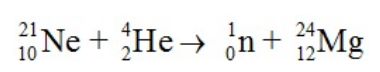# Problem: How much energy is released in the following nuclear reaction?Mass of the 21Ne = 20.993849 amu; Mass of 4He = 4.002604 amu;Mass of a neutron = 1.008665; Mass of 24Mg = 23.985045(a) 9.51 x 107 kJ.mol(b) 471 kJ.mol(c) 9.22 x 1010 kJ/mol(d) 7.65 x 104 kJ/mol(e) 2.47 x 108 kJ/mol

###### FREE Expert Solution
85% (417 ratings)
###### FREE Expert Solution

We’re being asked to calculate the energy released for the following nuclear reaction:

${}_{\mathbf{10}}{}^{\mathbf{21}}\mathbit{N}\mathbit{e}\mathbf{+}{}_{\mathbf{2}}{}^{\mathbf{4}}\mathbit{H}\mathbit{e}\mathbf{\to }{}_{\mathbf{0}}{}^{\mathbf{1}}\mathbit{n}\mathbf{+}{}_{\mathbf{12}}{}^{\mathbf{24}}\mathbit{M}\mathbit{g}$

To calculate the energy released for the reaction, we’re going to use the following steps:

Step 1: Calculate the mass defect (Δm).
Step 2: Calculate the mass defect (Δm) in kg.
Step 3: Calculate the energy released (E).

Step 1: Calculate the mass defect (Δm).

Given:

85% (417 ratings)###### Problem Details

How much energy is released in the following nuclear reaction?

Mass of the 21Ne = 20.993849 amu; Mass of 4He = 4.002604 amu;

Mass of a neutron = 1.008665; Mass of 24Mg = 23.985045

(a) 9.51 x 107 kJ.mol

(b) 471 kJ.mol

(c) 9.22 x 1010 kJ/mol

(d) 7.65 x 104 kJ/mol

(e) 2.47 x 108 kJ/molWhat scientific concept do you need to know in order to solve this problem?

Our tutors have indicated that to solve this problem you will need to apply the Mass Defect concept. If you need more Mass Defect practice, you can also practice Mass Defect practice problems.

What is the difficulty of this problem?

Our tutors rated the difficulty ofHow much energy is released in the following nuclear reactio...as high difficulty.

How long does this problem take to solve?

Our expert Chemistry tutor, Jules took 5 minutes and 7 seconds to solve this problem. You can follow their steps in the video explanation above.

What professor is this problem relevant for?

Based on our data, we think this problem is relevant for Professor Naleway's class at LUC.